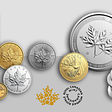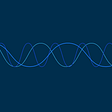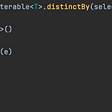# Comparing BigDecimals for Equality

I had some code that subtracted two BigDecimal numbers in Kotlin (the JVM version of Kotlin, that is), and I needed to check if the result was zero to avoid a divide-by-zero error. To do that, I tried simply comparing the result of the subtraction to a `BigDecimal` number with value zero, like so: `bdy - bdx == BigDecimal.ZERO` . As a simple test of this:

`val bdy = 0.3.toBigDecimal()val bdx = 0.3.toBigDecimal()println(bdy - bdx == BigDecimal.ZERO)`

This printed `false` , not what I expected.

Now, when you do arithmetic on floating point numbers (floats, doubles), their results won’t always be precise. For example, on my system if I were to add the doubles `0.2` and `0.1` (`println(0.2 + 0.1)`), the result isn’t exactly `0.3` (I get `0.30000000000000004` ). So maybe that’s what was going on here. But I was using `BigDecimal`, and arithmetic operations on BigDecimals are generally not prone to such imprecision issues like IEEE-754 floating point primitives. I decided to verify that by printing the result of subtracting my two BigDecimal numbers `bdy - bdx` , and sure enough the result was `0.0` . Furthermore, if I looked in the debugger at the value of `bdz = bdy - bdx` , the value of `bdz` was all zeroes as expected:

So then I decided to look at the Javadoc for the `==` (`equals()`) method for BigDecimal, and it said something interesting:

Unlike `compareTo`, this method considers two `BigDecimal` objects equal only if they are equal in value and scale (thus 2.0 is not equal to 2.00 when compared by this method).

So when I looked at the actual value of `BigDecimal.ZERO` in the debugger, it came up as this:

Note that one field in particular is different than the above: the `scale`. It’s `0` in this case, whereas above it’s `1` . Since they’re different, the `==` operation does not consider them to be equal.

Instead, the Javadoc suggests that `compareTo` be used, because in this case I don’t actually care about the scale used to represent the number; I only care that the operation’s value is zero, regardless of what scale is used to represent it.

Sure enough, when I changed `==` to `compareTo()` like so:

`val bdy = 0.3.toBigDecimal()val bdx = 0.3.toBigDecimal()println(BigDecimal.ZERO.compareTo(bdy - bdx) == 0)`

It printed out`true` .

This makes me wonder why the `equals()` method is implemented like this for `BigDecimal` . It seems odd to me that the former doesn’t work just because it’s picky about scale. I mean, I suppose there are some use cases where we might want to know if the scales are exact, but it seems that if we really care about that it would be better if we could do the something like `bdx == bdy && bdx.scale() == bdy.scale()` . Then again, I guess the`equals()` function for most classes tends to compare all fields, or at least all the “significant” ones. So by that argument I guess having `equals()`compare `scale` as well makes sense.

I guess it’s because in Kotlin we use the `==` operator rather than just write out the call to the`equals()`method like we would in Java code. And so it might not look as natural for this situation to fail because, when reading it in code, the `==`probably looks more like it would just compare values rather than doing the structural equality that `equals()` actually does.

Anyways, to summarize: use compareTo() when you only want to check if the values of two BigDecimals are equal.

--

--

--

## More from Brian Terczynski

Documenting my learnings on my journey as a software engineer.

Love podcasts or audiobooks? Learn on the go with our new app.

## Hackathons: our path to decentralization## PyQt Radio Buttons and the Mediator Pattern## Why 2020 should be the year you learn Rust?## The Other Ubi## Migrating to Java 11 while maintaining a Java 8 client library## Demand Forecasting## Brian Terczynski

Documenting my learnings on my journey as a software engineer.

## Kotlin Multiplatform library with Kotest and Gradle Version Catalog## Why KTS instead of Groovy?## Analyzing the algorithmic complexity of the Kotlin API’s distinctBy function## Caffeine cache simplified in Kotlin